# RD Sharma Solutions for Class 9 Maths Chapter 13 Linear Equations in Two Variables Exercise 13.4

RD Sharma Solutions for Class 9 Maths are given here for Chapter 13 exercise 13.4. This exercise deals with the equations of lines parallel to the x-axis and y-axis. Any point in the form (x, 0), where x is any real number, lies on x-axis. Because y-coordinate of every point on the x-axis is zero. The equation of x-axis is y=0. Similarly the equation of y-axis is x=0 as x-co-ordinate of every point on the y-axis is zero. Students can download RD Sharma Solutions class 9 chapter 13 exercise 13.3 and practice to score good marks in the examinations.

## Download PDF of RD Sharma Solutions for Class 9 Maths Chapter 13 Linear Equations in Two Variables Exercise 13.4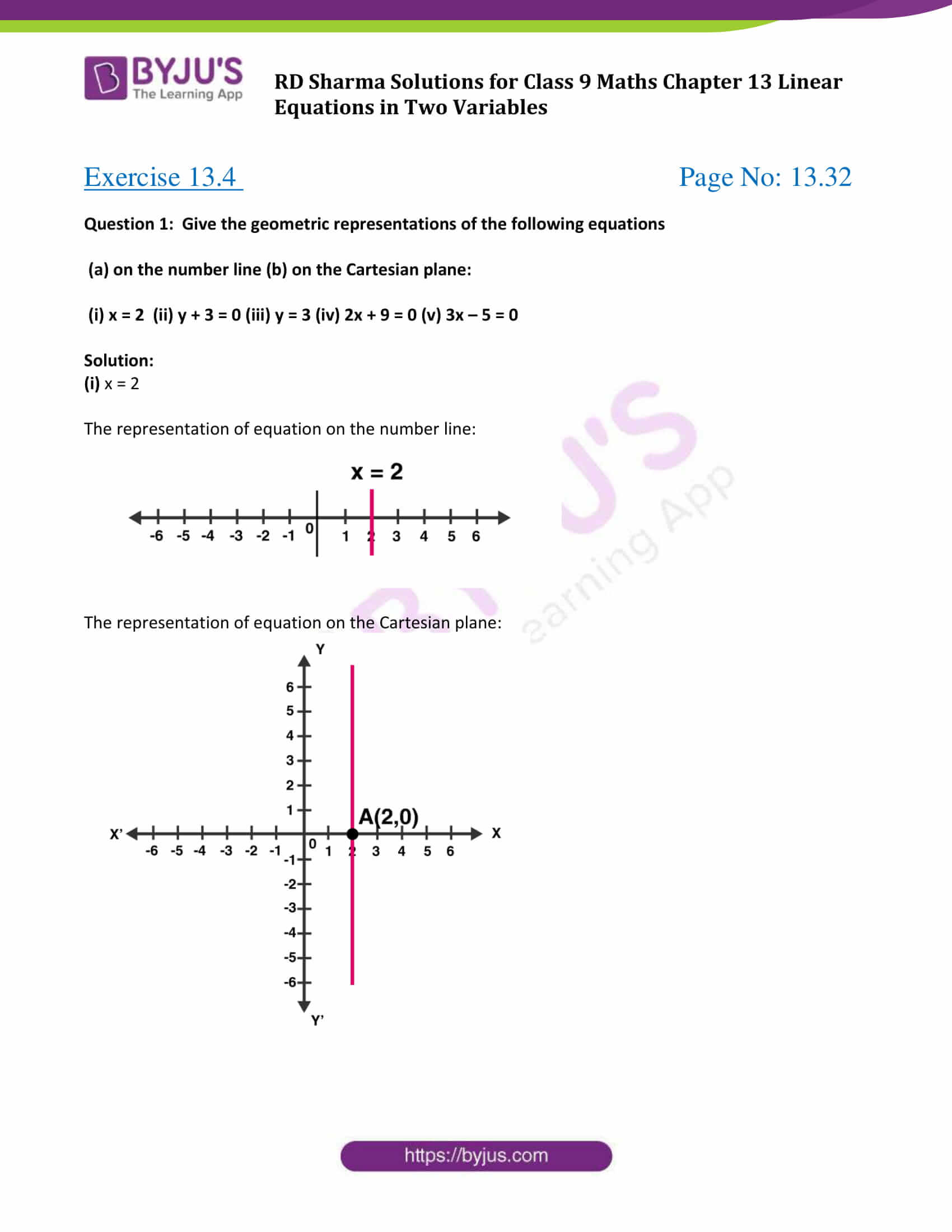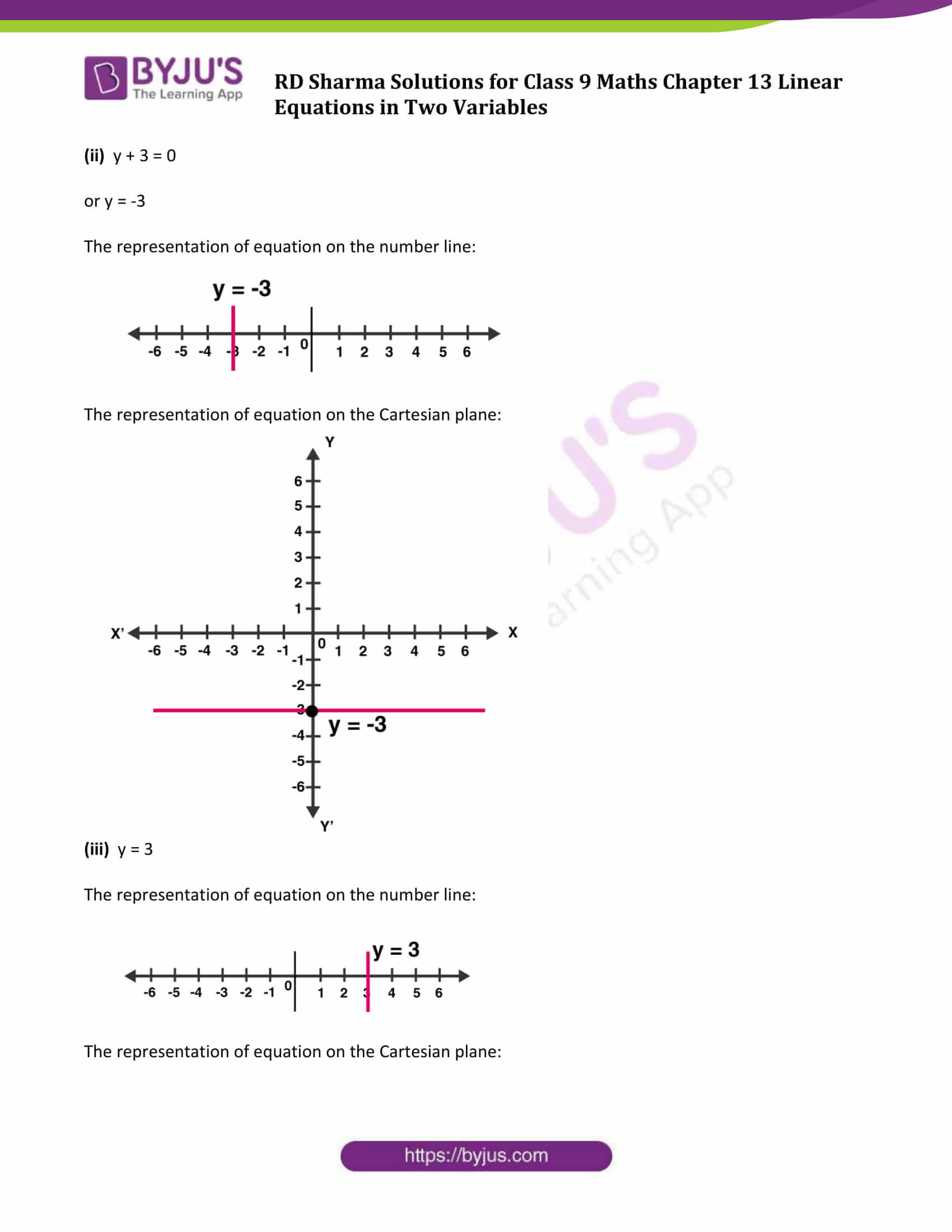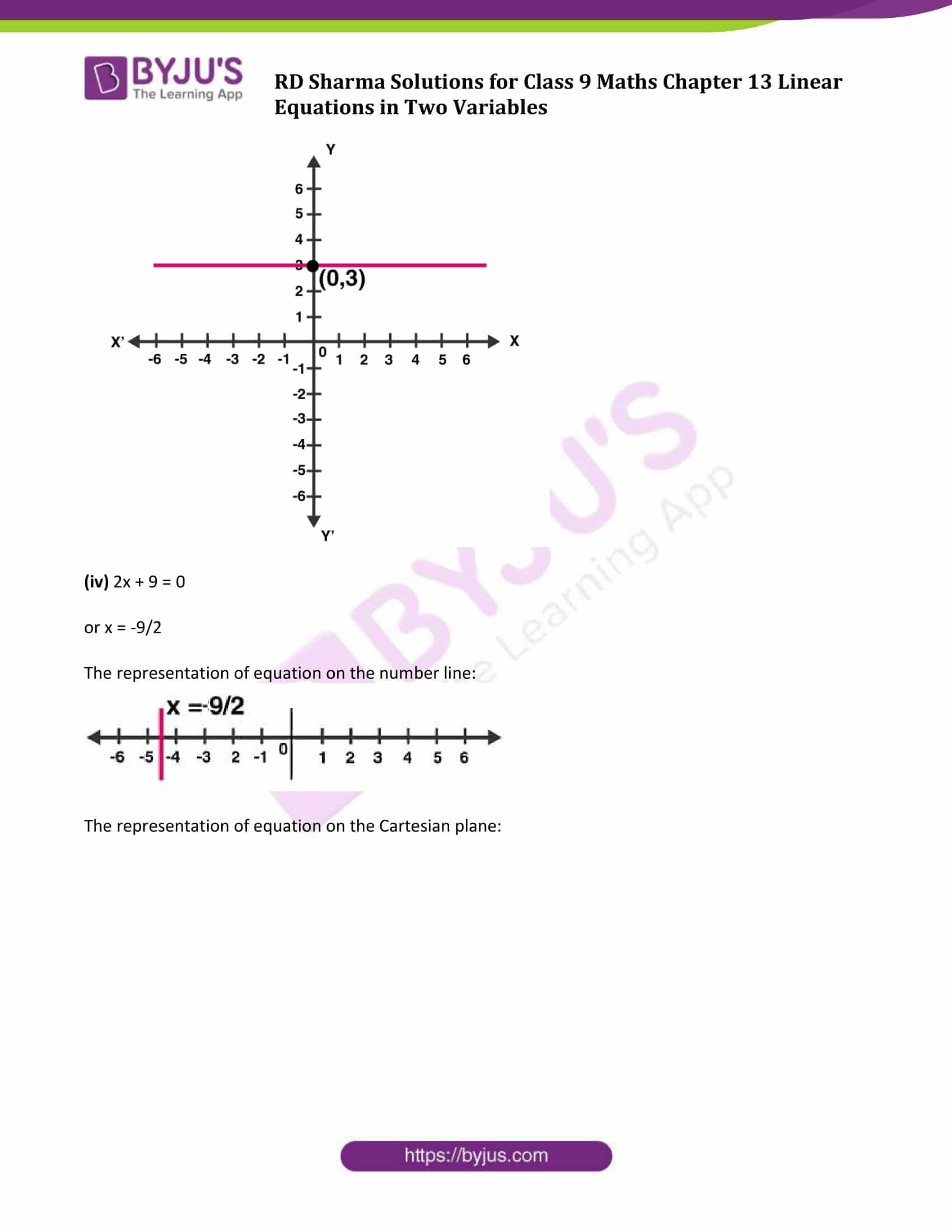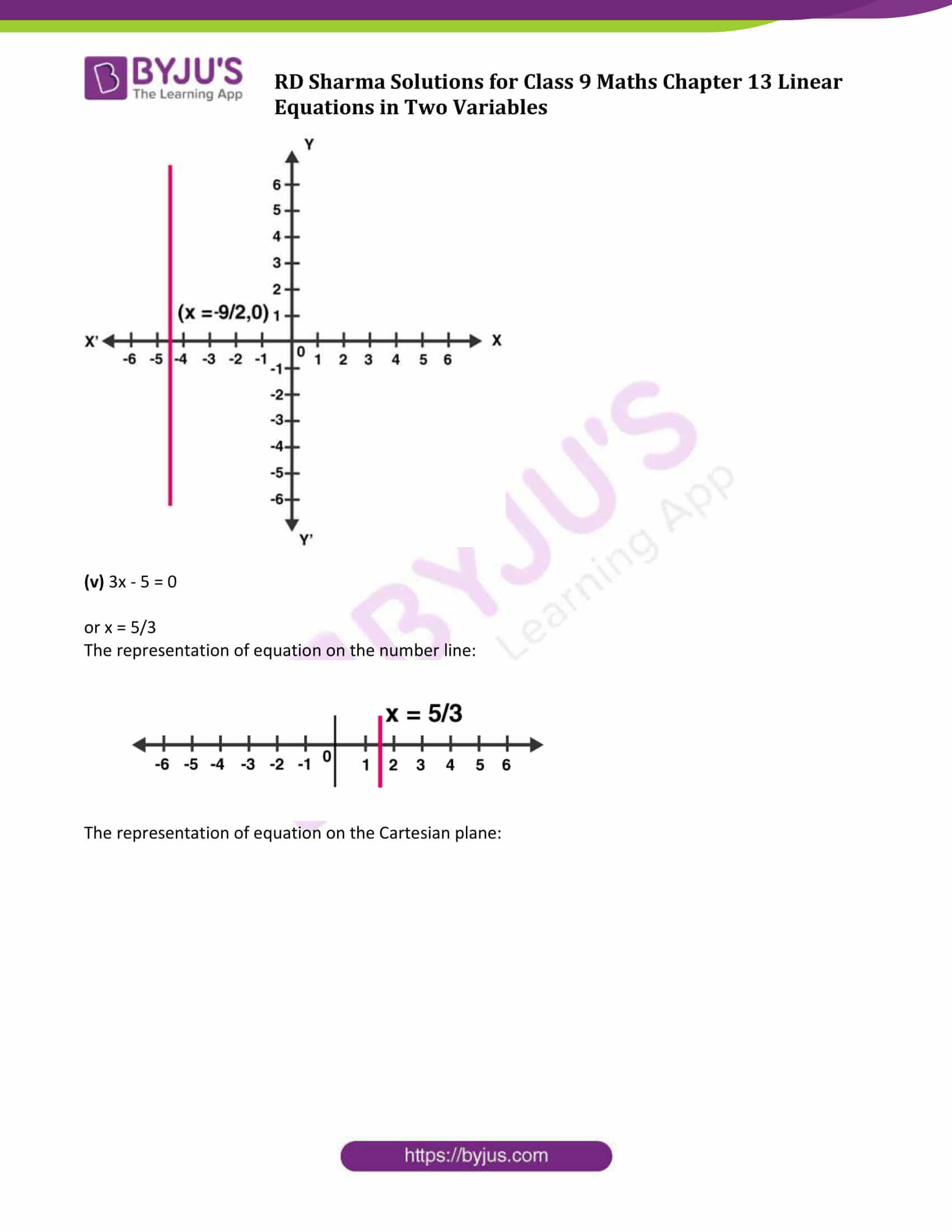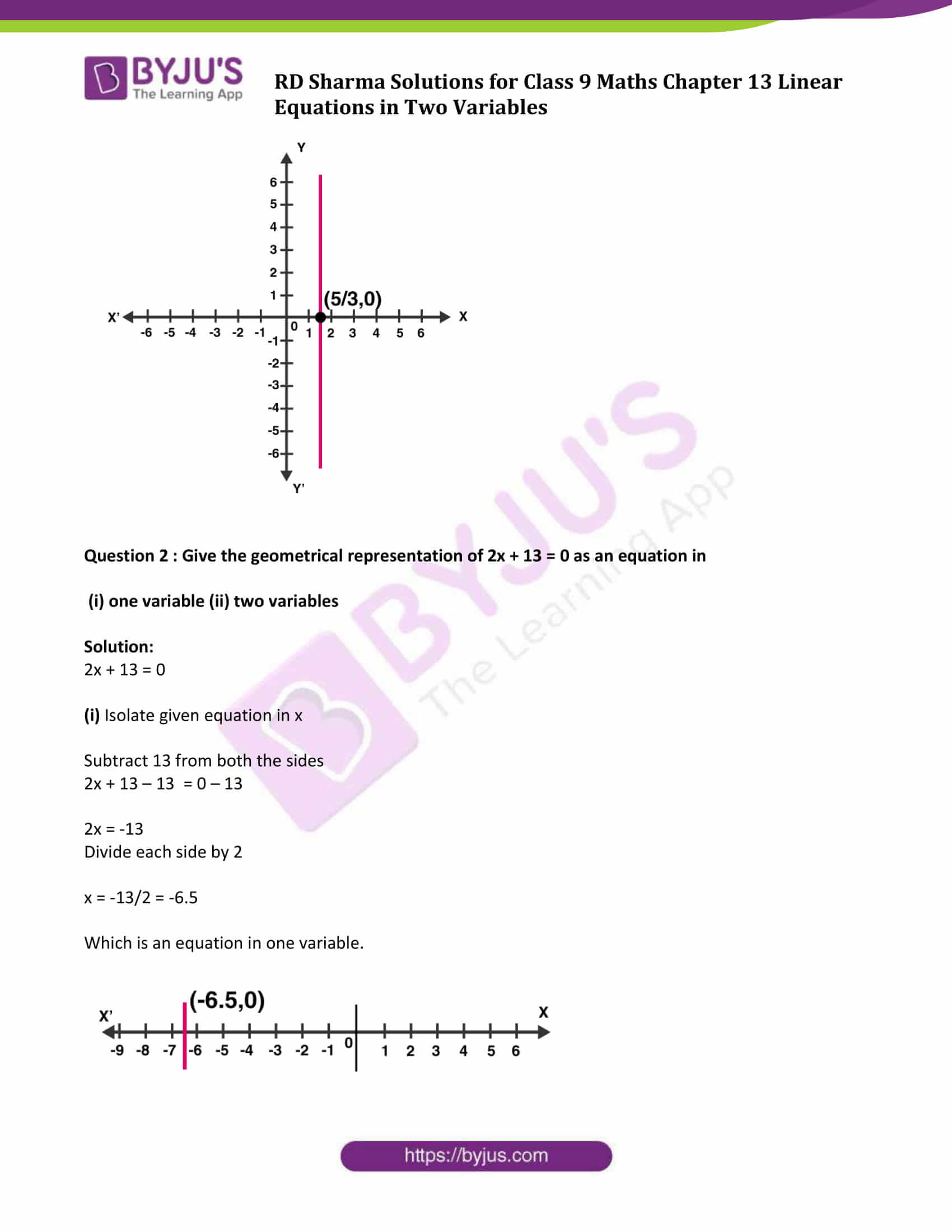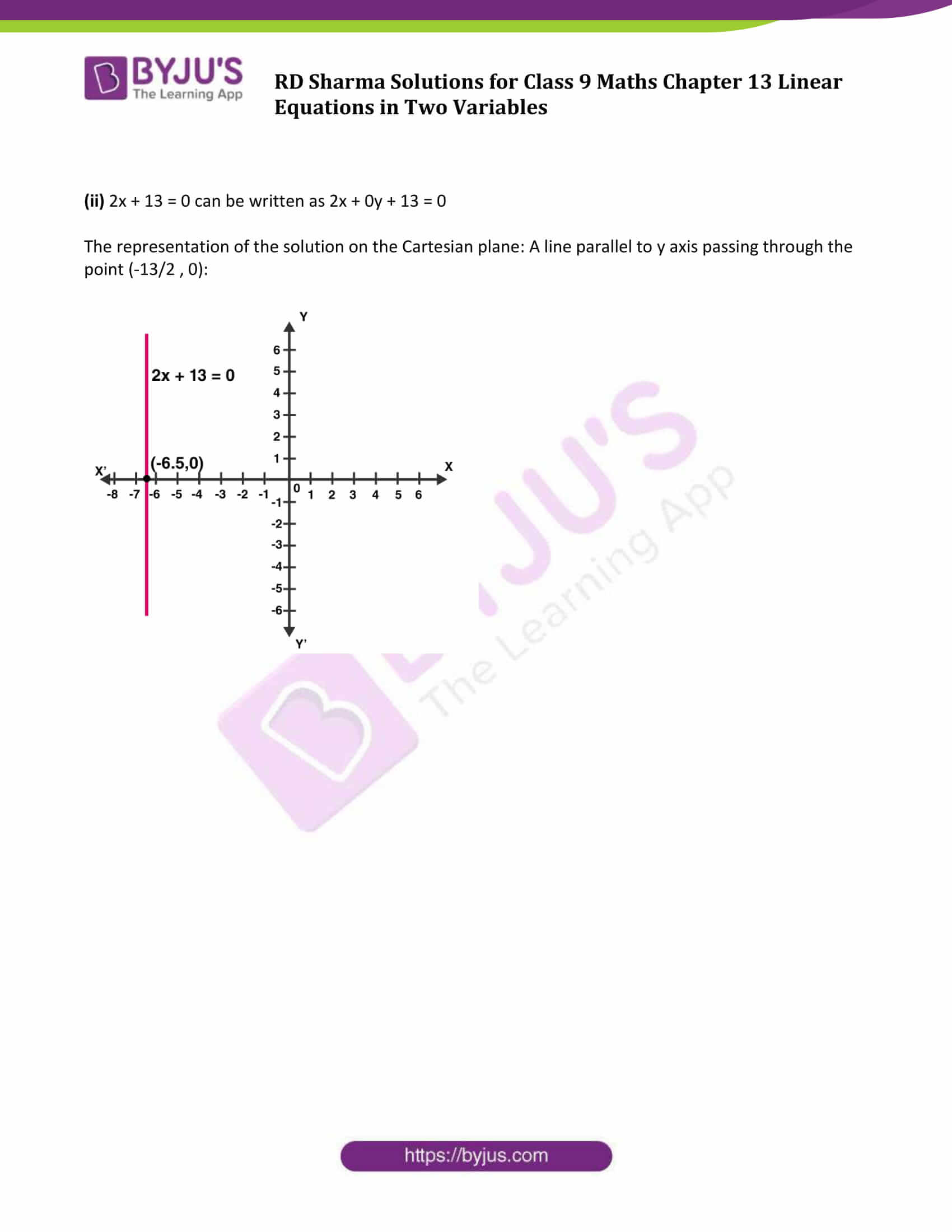### Access Answers to Maths RD Sharma Solutions for Class 9 Chapter 13 Linear Equations in Two Variables Exercise 13.4 Page number 13.32

Question 1: Give the geometric representations of the following equations

(a) on the number line (b) on the Cartesian plane:

(i) x = 2 (ii) y + 3 = 0 (iii) y = 3 (iv) 2x + 9 = 0 (v) 3x – 5 = 0

Solution:

(i) x = 2

The representation of equation on the number line: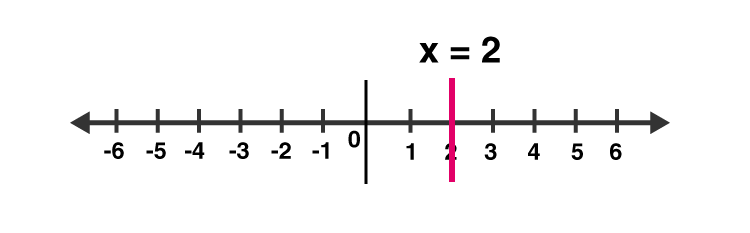The representation of equation on the Cartesian plane: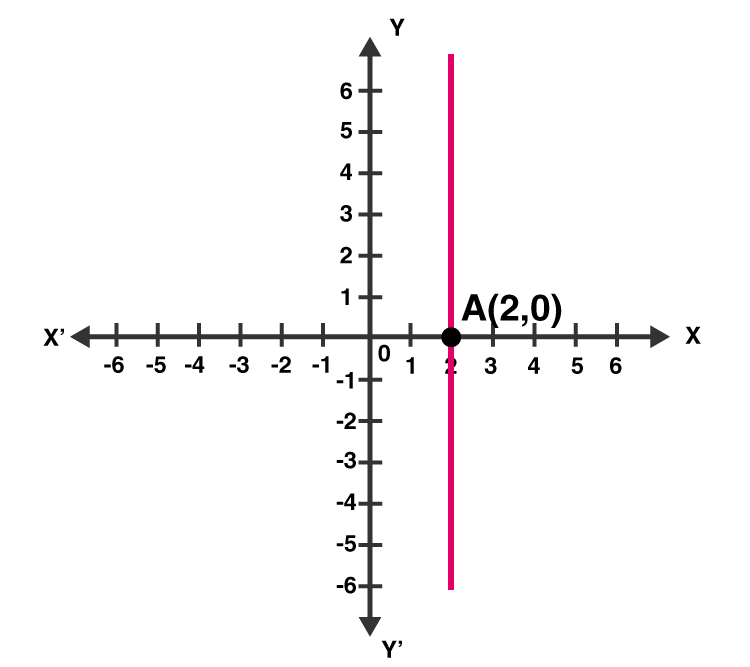(ii) y + 3 = 0

or y = -3

The representation of equation on the number line: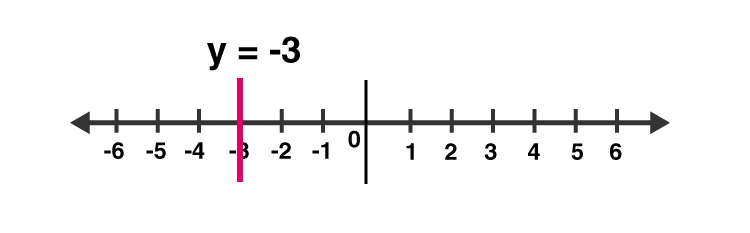The representation of equation on the Cartesian plane: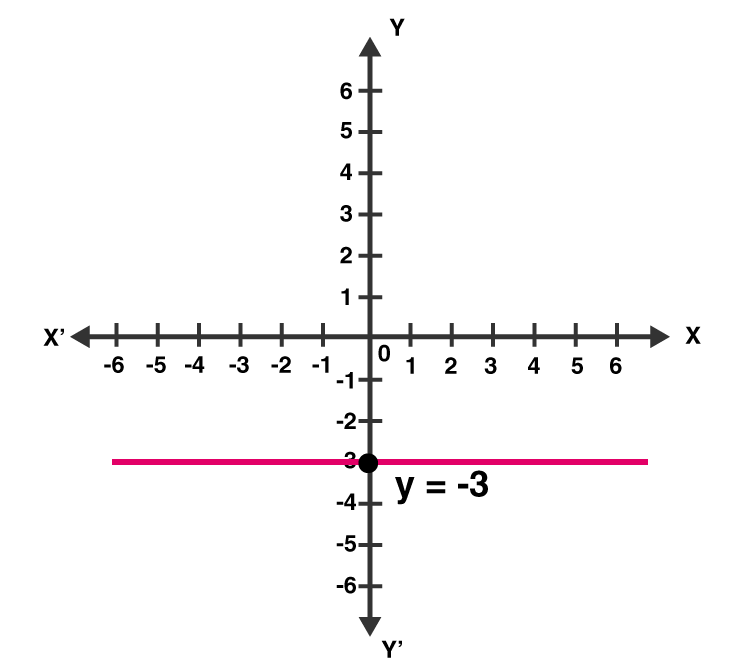(iii) y = 3

The representation of equation on the number line: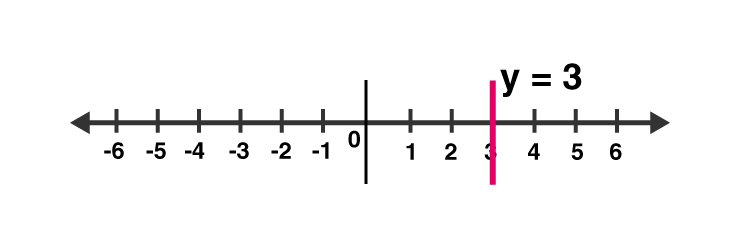The representation of equation on the Cartesian plane: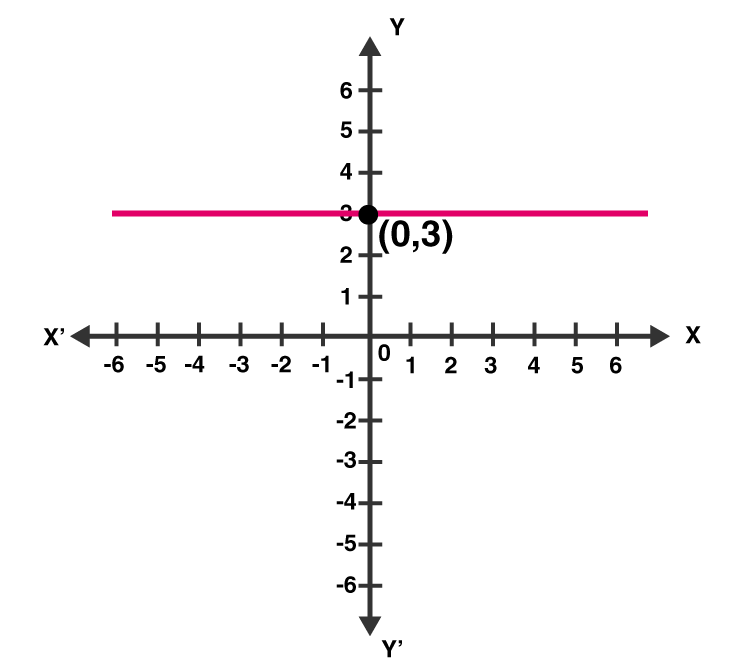(iv) 2x + 9 = 0

or x = -9/2

The representation of equation on the number line: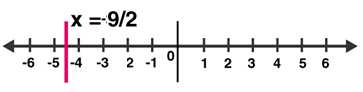The representation of equation on the Cartesian plane: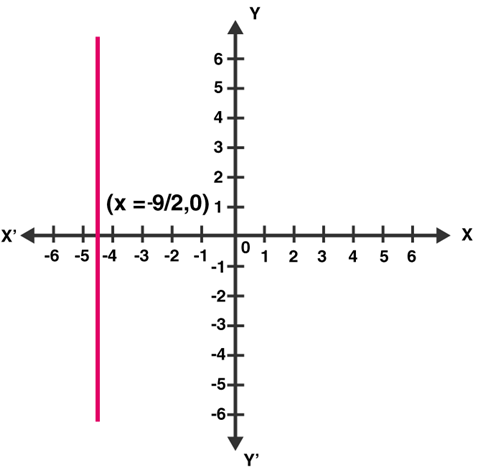(v) 3x – 5 = 0

or x = 5/3

The representation of equation on the number line: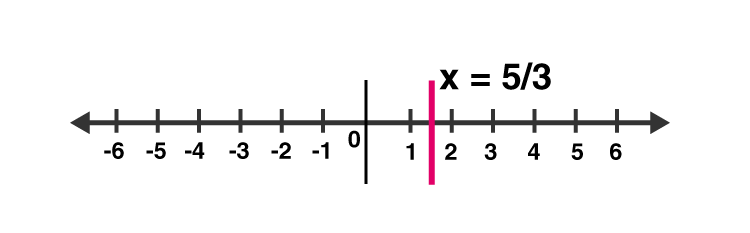The representation of equation on the Cartesian plane: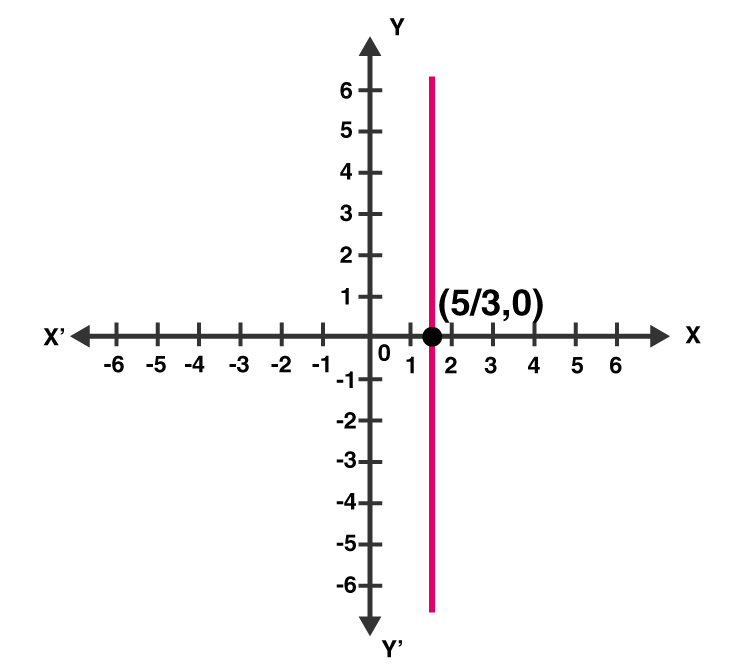Question 2 : Give the geometrical representation of 2x + 13 = 0 as an equation in

(i) one variable (ii) two variables

Solution:

2x + 13 = 0

(i) Isolate given equation in x

Subtract 13 from both the sides

2x + 13 – 13 = 0 – 13

2x = -13

Divide each side by 2

x = -13/2 = -6.5

Which is an equation in one variable.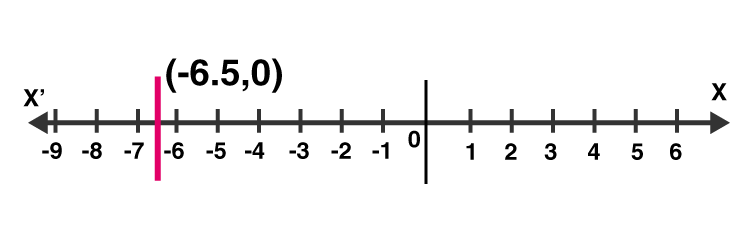(ii) 2x + 13 = 0 can be written as 2x + 0y + 13 = 0

The representation of the solution on the Cartesian plane: A line parallel to y axis passing through the point (-13/2 , 0):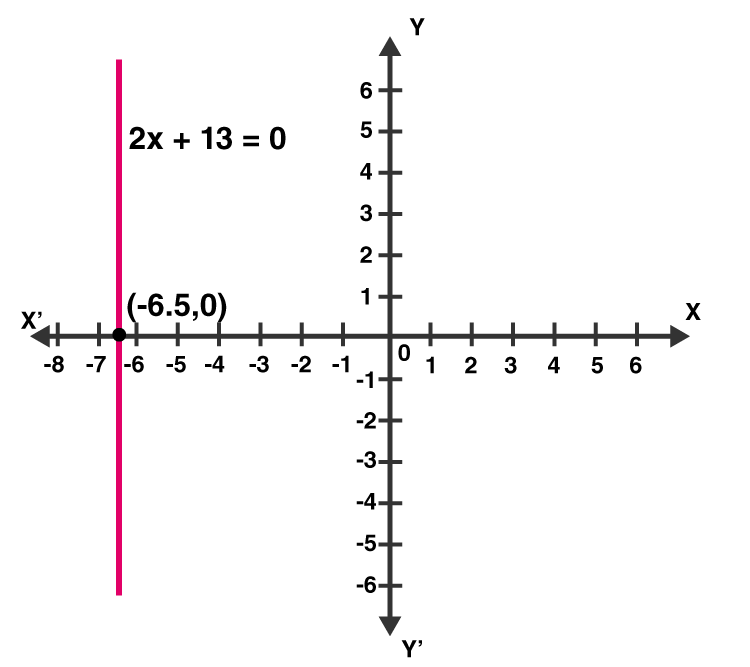## RD Sharma Solutions for Class 9 Maths Chapter 13 Linear Equations in Two Variables Exercise 13.4

RD Sharma Solutions Class 9 Maths Chapter 13 Linear Equations in Two Variables Exercise 13.4 is based on topic – Equations of lines parallel to the x-axis and y-axis. Students can download RD Sharma chapter 13 exercise 13.4 solutions and practice to score good marks in the examinations.